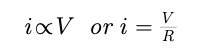# Physics - What is Ohm's Law?

Ohm's Law

At constant temperature, the current passing through a conductor is proportional to the potential difference between its ends.If the potential difference between the ends of a Conductor AB is V and the current through it is i, then according to Ohm's Law,where R is a constant known as Electrical Resistance of the Conductor.

Limitations of Ohm's Law

Ohm's law is applicable to metal conductors when physical constants dimensions and temperature are constant.

The Conductors that obey ohm's law are called ohmic registors.

E.g Metals

Post By : Rahul Kumar 04 Jun, 2020 466 views Physics